# zerocrossrate

Zero-crossing rate

## Syntax

``rate = zerocrossrate(x)``
``rate = zerocrossrate(TT)``
``rate = zerocrossrate(___,Name,Value)``
``[rate,count] = zerocrossrate(___)``
``[rate,count,indices] = zerocrossrate(___)``
``zerocrossrate(___)``

## Description

example

````rate = zerocrossrate(x)` returns the zero-crossing rate of `x`. If `x` is a matrix, then the function analyzes each column as a separate channel and returns the zero-crossing rate as a row vector where each value corresponds to a channel.```

example

````rate = zerocrossrate(TT)` returns the zero-crossing rate of the data stored in the MATLAB® timetable `TT`. If `TT` contains multiple channels, then the function analyzes each channel independently.```

example

````rate = zerocrossrate(___,Name,Value)` specifies additional name-value arguments. Use this syntax with any of the input arguments in previous syntaxes.```

example

````[rate,count] = zerocrossrate(___)` also returns the total number of crossings in `count`.```

example

````[rate,count,indices] = zerocrossrate(___)` also returns logical indices at the signal locations where a crossing occurs.```
````zerocrossrate(___)` with no output arguments plots `rate` along the y-axis and the corresponding window number along the x-axis. If the window length is equal to the full signal length, then the function plots the length of the window along the x-axis and the crossing rate in the middle of the window.```

## Examples

collapse all

Consider a vector of ones with alternating signs. Plot the data.

```x = [1 -1 1 -1 1 -1 1 -1 1 -1]; plot(x)```Compute the zero-crossing rate of `x`.

`r = zerocrossrate(x)`
```r = 0.9500 ```

Use the third output argument to find the locations where the crossings occur. Plot `x` and the zero-crossing locations. The function returns an index at the next sample after a crossing, not necessarily the exact crossing location. The first sample is marked as a crossing point because the function considers the initial state of `x` to be zero by default.

```[~,~,indices] = zerocrossrate(x); plot(x) hold on plot(x(indices),'*') hold off```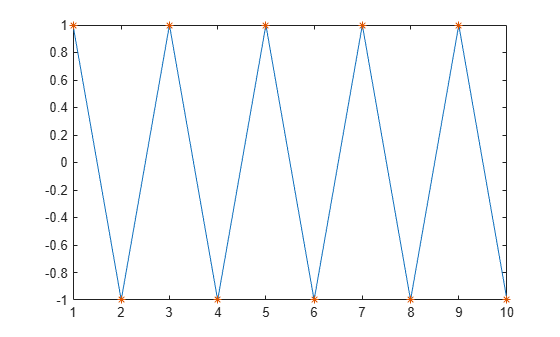Compute the zero-crossing rate of `x` using the comparison method. The rate differs from the value computed using the difference method.

`rC = zerocrossrate(x,Method="comparison")`
```rC = 0.9000 ```

Compute the zero-crossing rate of `x` again using the difference method and specify zero as positive. The rate is equal to the value computed using the comparison method.

`rZ = zerocrossrate(x,ZeroPositive=1)`
```rZ = 0.9000 ```

Now specify the initial state of `x` as `1`. The rate is equal to the previous result.

`rI = zerocrossrate(x,InitialState=1)`
```rI = 0.9000 ```

Load a set of temperature readings in Celsius taken every hour at Logan Airport in Boston for the entire month of January, 2011. Create a `timetable` and use `retime` to aggregate the data into daily means.

```load bostemp t = hours(1:24*31)'; TT = timetable(t,tempC); rTT = retime(TT,'daily','mean');```

Count the number of days the temperature crosses the monthly average. Plot the data and include a horizontal line at the monthly average temperature to visualize where the crossings occur.

`avg = mean(TT.tempC)`
```avg = -1.3007 ```
`[~,count] = zerocrossrate(rTT,Level=avg)`
```count = 9 ```
```plot(hours(rTT.t/24),rTT.tempC) yline(avg) xlabel('Time elapsed since January 1, 2011 (days)') ylabel('Average daily temperature (\circC)') axis tight```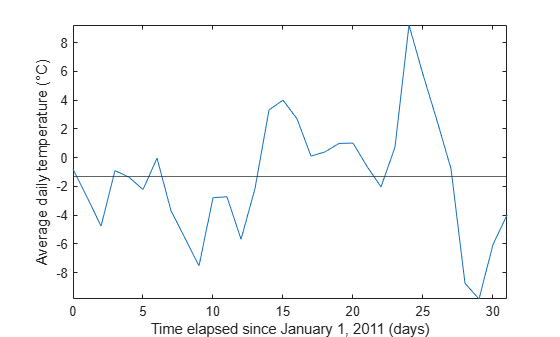Speech can be characterized as being voiced or unvoiced. Voiced speech, such as vowel sounds, occurs when the vocal cords vibrate. In unvoiced speech, such as most consonant sounds, the vocal chords do not vibrate. You can use zero crossings to classify the voiced and unvoiced regions in an audio signal.

Load an audio signal into the MATLAB® workspace. The voice says, "Oak is strong, and also gives shade".

```[y,fs] = audioread("oak.m4a"); % To hear, type soundsc(y,fs)```

The signal is sampled at 44.1 kHz. Calculate the zero-crossing rate for 10 ms windows using the comparison method.

```win = fs*0.01; rate = zerocrossrate(y,WindowLength=win,Method="comparison");```

Plot `rate` to visualize the crossing rate for each segment. Voiced speech is expected to have a low crossing rate, while unvoiced speech is expected to have a high crossing rate.

`plot(rate)`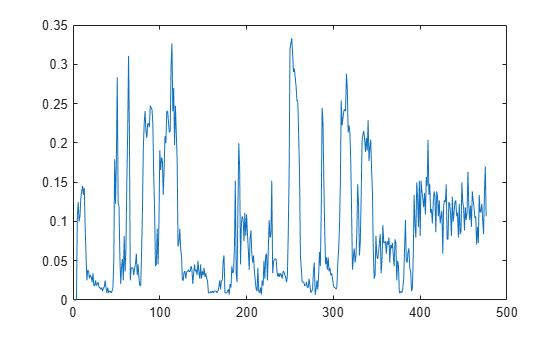Use a threshold of `0.1` to differentiate between voiced and unvoiced segments. Create a `signalMask` object that has two categories ("Unvoiced" and "Voiced") and plot the regions of interest (ROIs). Compare the regions of voiced and unvoiced speech to the location of each spoken word.

IBM® Watson Speech to Text API and Audio Toolbox™ software can be used to extract words from an audio file. Load T`ranscription.mat` into the workspace. The labeled signal set contains the audio signal, ROI limits, and labels for each spoken word. For details, see Label Spoken Words in Audio Signals. Display the spoken words on the plot.

```h = 0.1; idu = find(rate > h); idu(1:2) = []; vi = [(idu-1) idu]*win; m = sigroi2binmask(vi,length(y)); mask = signalMask([m ~m],Categories=["Unvoiced" "Voiced"],SampleRate=fs); plotsigroi(mask,y) load Transcription ln = getLabelNames(transcribedAudio); v = getLabelValues(transcribedAudio,1,ln); v.Value = categorical(v.Value,v.Value); RL = v.ROILimits; VL = v.Value; hold on text(mean(RL,2),-0.7*ones(size(VL)),VL,HorizontalAlignment="center", ... FontSize=11,FontWeight="bold") hold off```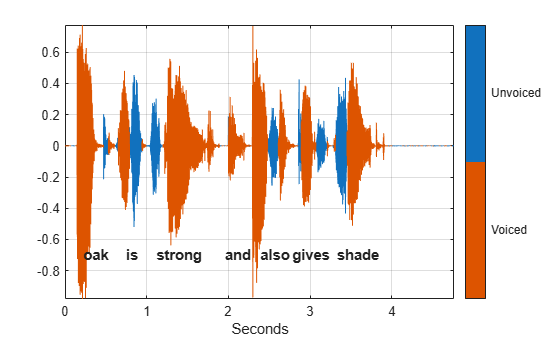Load an audio file containing 15 seconds of acoustic guitar music. The sample rate is 44.1 kHz. To play the music, uncomment the last line of code.

```Fs = 44100; y = audioread("guitartune.wav"); % sound(y,Fs)```

Buffer the audio signal into overlapping frames, each with 4096 samples. Use an overlap of 512 samples.

```winLength = 4096; overlap = 512; [yB,~] = buffer(y,winLength,overlap,"nodelay");```

Obtain the zero-crossing rate for each frame. To account for frame overlap, specify the initial state as the previous value of the first sample in each frame.

```init = winLength - overlap; state = 0; zcr = []; for i = 1:size(yB,2) zcr = [zcr;zerocrossrate(yB(:,i),InitialState=state)]; state = yB(init,i); end```

Plot the audio signal and overlay the zero-crossing rate for each frame.

```figure yyaxis left x = 0:1/Fs:(numel(y)-1)/Fs; plot(x',y) xlabel("Seconds") ylabel("Amplitude") yyaxis right xx = (1:size(yB,2))*((winLength-overlap)/Fs); plot(xx',zcr) ylabel("Zero-crossing rate")```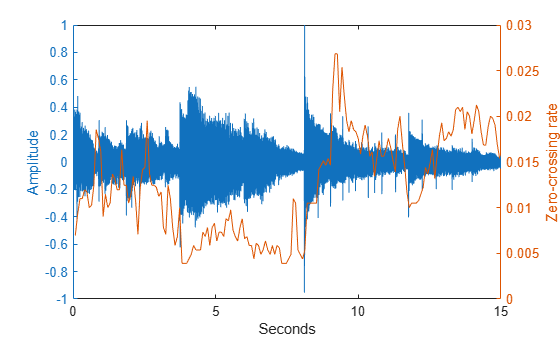Compute the zero-crossing rate of the unbuffered signal. To obtain a result equivalent to the zero-crossing rate of the buffered signal, set `WindowLength` to `4096` and `OverlapLength` to `512`. Determine if the two results are equal.

```zcr_batch = zerocrossrate(y,WindowLength=winLength,OverlapLength=overlap); isequal(zcr_batch,zcr)```
```ans = logical 1 ```

## Input Arguments

collapse all

Data, specified as a real-valued vector or matrix. If `x` is a matrix, the function returns the zero-crossing rate as a row vector where each value corresponds to a column of data.

Data Types: `single` | `double`

Input timetable, specified as a `timetable`. `TT` must contain uniformly sampled single- or double-precision data. The RowTimes property must contain a `duration` or `datetime` vector with increasing and finite values. If `TT` is a timetable with a single variable containing a matrix, or a timetable with multiple variables each containing a vector, then the function analyzes each channel independently.

### Name-Value Arguments

Specify optional pairs of arguments as `Name1=Value1,...,NameN=ValueN`, where `Name` is the argument name and `Value` is the corresponding value. Name-value arguments must appear after other arguments, but the order of the pairs does not matter.

Before R2021a, use commas to separate each name and value, and enclose `Name` in quotes.

Example: `zerocrossrate(x,Method="comparison",Level=7,transitionEdge="rising")` uses the comparison method to compute the rate at which `x` positively transitions across `7`.

Previous states of `x`, specified as a vector whose number of elements is equal to the number of input channels.

Example: `zerocrossrate(x,InitialState=[1 0 –1 3])` returns the crossing rates of a four-channel input signal `x`.

Data Types: `single` | `double` | `int8` | `int16` | `int32` | `int64` | `uint8` | `uint16` | `uint32` | `uint64`

Method for computing the zero-crossing rate, specified as `"difference"` or `"comparison"`. If you do not specify `Method`, the function uses the difference method to compute the crossing rate.

• `comparison` — The function marks the `indices` as true where a crossing is fully completed.

• `difference` — The function marks the `indices` as true where abs(sign(xi)–sign(xi–1)) > 0.

Example: `zerocrossrate(x,Method="comparison")` computes the crossing rate of `x` using the comparison method.

Data Types: `char` | `string`

Window length over which to compute the crossing rate, specified as a positive integer. The default window length is the signal length.

Example: `zerocrossrate(x,WindowLength=20)` returns the crossing rates for 20-sample windows in `x`.

Example: `zerocrossrate(x,WindowLength=fs*0.05)` returns the crossing rates for 50 ms windows in `x` given a sample rate `fs`.

Data Types: `single` | `double` | `int8` | `int16` | `int32` | `int64` | `uint8` | `uint16` | `uint32` | `uint64`

Number of overlapping samples between adjoining segments, specified as a positive integer. The overlap must be smaller than the window length.

Example: `zerocrossrate(x,OverlapLength=0)` returns the crossing rates of segments with no overlap.

Example: `zerocrossrate(x,WindowLength=20,OverlapLength=5)` returns the crossing rates of overlapping segments with five samples of overlap.

Data Types: `single` | `double` | `int8` | `int16` | `int32` | `int64` | `uint8` | `uint16` | `uint32` | `uint64`

Signal level for which the crossing rate is computed, specified as a real scalar. The function subtracts the `Level` value from the signal and then finds the zero crossings. If you do not specify `Level`, the function uses the default value of `0` and returns the zero-crossing rate.

Example: `zerocrossrate(x,Level=1)` returns the rate at which the input signal `x` crosses `1`.

Data Types: `single` | `double` | `int8` | `int16` | `int32` | `int64` | `uint8` | `uint16` | `uint32` | `uint64`

Threshold value above and below the `Level` value over which the crossing rate is computed, specified as a real scalar. The function sets all the values of the input in the range [–`threshold`, `threshold`] to `0` and then finds the zero crossings.

Example: `zerocrossrate(x,Threshold=0.1)` returns the crossing rate with a tolerance of –0.1 to 0.1.

Note

When you specify both `Level` and `Threshold`, the function first subtracts the level value from the input and then sets to `0` the resulting input values that are in the range [–`threshold`, `threshold`].

Data Types: `single` | `double` | `int8` | `int16` | `int32` | `int64` | `uint8` | `uint16` | `uint32` | `uint64`

Transitions to include when counting the zero crossings, specified as `"falling"`, `"rising"`, or `"both"`. If you specify `"falling"`, the function counts only negative-going transitions. If you specify `"rising"`, the function counts only positive-going transitions.

Example: `zerocrossrate(x,TransitionEdge="rising")` returns the crossing rate of `x` for only positive-going transitions.

Data Types: `char` | `string`

Sign convention, specified as a logical scalar. If you specify `ZeroPositive` as true, the function considers `0` to be positive. If you specify `ZeroPositive` as false, the function considers `0`, –`1`, and +`1` to have distinct signs following the convention of the `sign` function.

Example: `zerocrossrate(x,ZeroPositive=1)` returns the crossing rate of the input signal `x` and considers zero as positive.

Data Types: `logical`

## Output Arguments

collapse all

Zero-crossing rate, returned as a row vector or a matrix. When `WindowLength` is equal to the signal length, `rate` is a row vector whose number of elements is equal to the number of channels in `x` or `TT`. When `WindowLength` is smaller than the signal length, the function returns `rate` as a matrix where the i-th row contains the crossing rate for the i-th window and the j-th column corresponds to the j-th input channel.

Number of crossings, returned as an N-by-M matrix where N is the number of windows and M is the number of input channels. The i-th row corresponds to the crossing count for the i-th window and the j-th column corresponds to the crossing count for the j-th channel.

Logical indices at the signal locations where crossings occur, returned as an N-by-`WindowLength`-by-M array where N is the number of windows and M is the number of input channels.

Note

Indices might not represent exact signal crossing locations. The `zerocrossrate` function returns an index at the next sample following a crossing.

## Version History

Introduced in R2021b